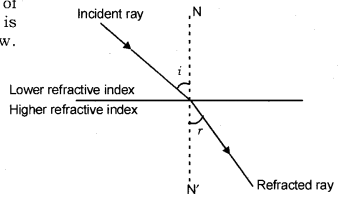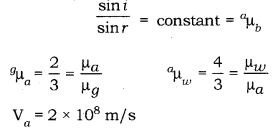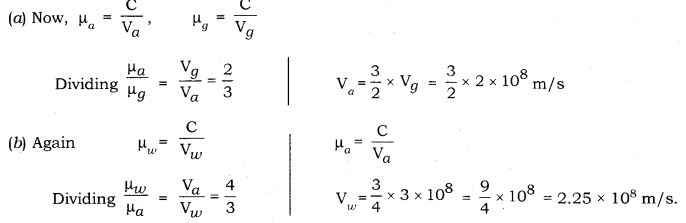# With the help of a ray diagram, state what is meant by refraction of light

With the help of a ray diagram, state what is meant by refraction of light. State Snell’s law for refraction of light and also express it mathematically.
The refractive index of air with respect to glass is 2/3 and the refractive index of water with respect to air is 4/3. If the speed of light in glass is 2 x 108 m/s, find the speed of light in
(a) air
(b) water.

When a ray of light travels from one transparent medium to another, it bends at the surface. This happens because different media have different optical densities.

The phenomenon of bending of light as it travels from one medium to another is known as refraction of light.

As a ray of light moves from an optically rarer medium to an optically denser medium, it bends towards the normal at the point of incidence. Therefore, the angle of incidence (i) is greater than the angle of refraction ®. Hence, i > r. The first law of refraction is also known as Snell’s law.

Snell’s law: The ratio of the sine of the angle of incidence to the sine of the angle of refraction is constant. This is known as Snell’s law. Mathematically, it can be given as follows:Now, we know μ = C/V
where μ is absolute
refractive index of a medium w.r.t. vacuum C is speed of light in vacuum, V is speed of light in medium.